;nbsp

Hi parents and students, welcome to Algebra 2! My courses cover all of the common core requirements (the syllabus for semester 1 is here, and semester 2 is here!) and prepare my students to think critically. Not only do I want your student to know the material in my class, I want them to succeed in all future classes and opportunities.

Use the following pacing chart to stay on track. Click individual lecture videos to make up work or stay ahead of the material!

Week Day A Day B
Week 1 10.1
10.2
10 Quiz
10 Review
Unit 10 Test
11.1
11 Quiz
11.2
11.3
Week 2 11.4
11.5
11 Review
Unit 11 Test
12.1
12.2
12.3
12.4
Week 3 12.5
12 Review
Unit 12 Test
14.1
14.2
14 Quiz
14.3
14 Review
Unit 14 Test
Week 4 15.1
15.2
15.3
15 Quiz
15.4
15.5
15.6
15 Review
Week 5 15 Test
16.1
16.2
16 Quiz
16.3
16.4
16.5
16.6
Week 6 16.7
16 Review
Unit 16 Test
17.1
17.2
17.3
17 Quiz
17.4
Week 7 17 Review
Unit 17 Test
18.1
18.2
18 Quiz
18.5
18.6
18 Review
Week 8 18 Test
Semester Review
Final

The following sample assessment is similar to your Algebra 2A final. You will be capable of solving all of these problems at the end of the class. This test auto corrects and sends immediate feedback if you’d like to gauge your abilities before the class. Try not to guess!

Name*

This is a required question

Email*

Must be a valid email address
This is a required question

1. Find the equation of the line parallel to the line with equation [ 3y = 6 ] and passing through (–1, 5).
This is a required question

2. Identify the equation of a parabola with vertex (1, -4) passing through (2, -2)
This is a required question

3. Is (1, -3) is part of the solution of [ 2x – y < 5 ]
Determine algebraically!
This is a required question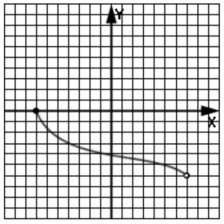4a. What is the domain of this graph?
This is a required question

4b. What is the range of this graph?
This is a required question

4c. Is this a function?
This is a required question

5. Expand [ (2y – 5)*(3y + 4) ]
This is a required question

6. Identify the solution of [ -2(x + 5) > 3x ] on a number line.
Solve for x and graph.
This is a required question7a. Five times the number of nerds is 9 more than half the nerds. What equation would you use to solve for n?
n = # of nerds
This is a required question

7b. How many nerds are there?
This is a required question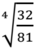8. Simplify [ ⁴√(32/81) ] and leave in simplest radical form.
This is a required question

9. Solve [ (5x – 2)^(3/5) = 27 ]
This is a required question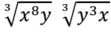10. Simplify [ (³√x⁸y)*(³√y³x) ]
This is a required question

11. Are [ f(x) = 1/2x – 5 ] and [ g(x) = 2x + 10 ] inverses of each other?
This is a required question

12. Identify f⁻¹(x) when f(x) = x – 9
This is a required question

13. Factor [ a² – 1/b² ]
This is a required question

14. In the function [ g(f) = 3f² – 2f + 9 ], which is the dependent variable?
This is a required question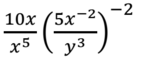15. Simplify [ (10x/x⁵)*(5x⁻²/y³)⁻² ]
This is a required question

16. Express (xy)^(3/5) in radical form.
This is a required question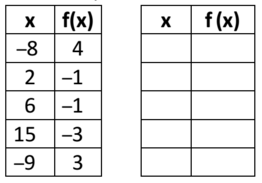17. The table on the right is filled in so that it represents a reflection of the left table across the y-axis. Identify one of the points in table 2.
This is a required question

18. Express [ 6zx ³√(xz⁴) ] in simplest exponential form.
This is a required question

19. Factor [ x² – 11x – 12 ]
This is a required question

20. Identify the correct intermediate formula when solving [ 3x² – 9x + 1 = 0 ] by completing the square.
This is a required question

21. Solve [ 3x² – 9x + 1 = 0 ] using the quadratic formula.
This is a required question

22. Find the equation of the line passing through (1,4) and (-2, -1)
This is a required question

23. Factor [ 24x² – 18xy + 3y² ]
This is a required question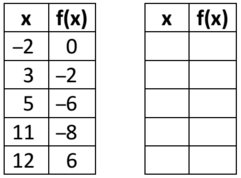24. The table on the right is filled in so that it represents a translation 3 units down and 1 unit to the right of the left table. Identify one of the points in table 2.
This is a required question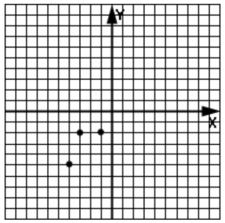25. Identify the graph that would make this an odd function.
This is a required question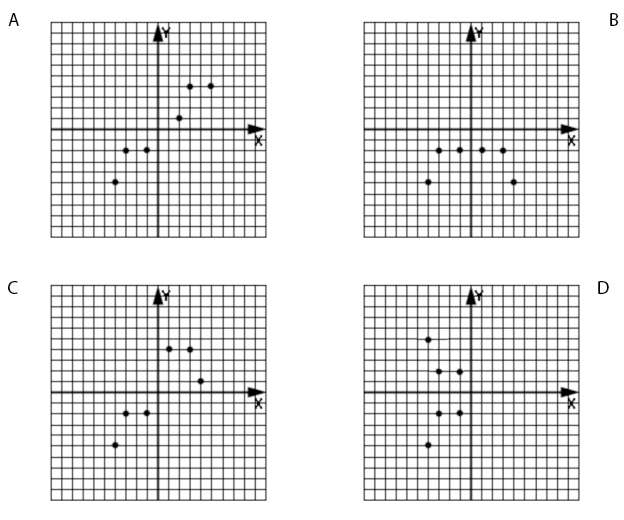26a. Five times the number of quarters is two more than the number of nickels. The total value of the coins is \$1.90. What system of equations would be used to solve for the number of coins?
Q = # of quarters, N = # of nickels
This is a required question

26b. How many quarters are there?
This is a required question

This is a required question

## 28. f(x) = 2(x + 4)² – 8

28a. What is the vertex of this quadratic function?
This is a required question

28b. What is the axis of symmetry?
This is a required question

28c. What is the maximum or minimum value of the function?
This is a required question

28d. What is the domain of the function?
This is a required question

28e. What is the range of the function?
This is a required question

28f. What is the y-intercept of the function?
This is a required question

28g. What are the roots of the function?
This is a required question

28h. Identify the graph of the function.
This is a required question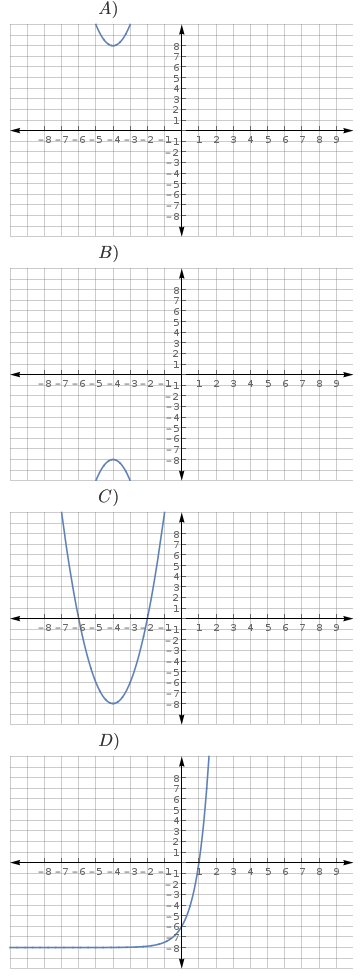29. Solve [ 7x² – 21 = 0 ]
Square root
This is a required question

30. Find f(3) where [ f(x) = 2x² – x + 5 ]
This is a required question

31. Solve the system of equations for [ 6x + y = 3 ] and [ 4x – 8 + y = 0 ]
Substition
This is a required question

32. Solve [ 4 + 2√(2x + 1) = 0 ]
This is a required question

33. Solve and graph the solution to [ 20 ≤ 4x ≤ 44 ] on a number line.
This is a required question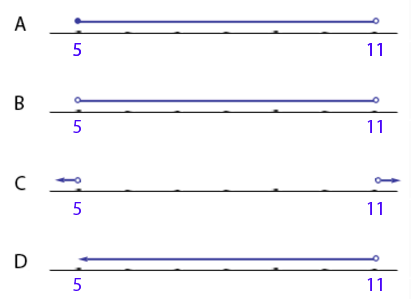This is a required question